MathScore EduFighter is one of the best math games on the Internet today. You can start playing for free!

## Positive Number Line - Sample Math Practice Problems

The math problems below can be generated by MathScore.com, a math practice program for schools and individual families. References to complexity and mode refer to the overall difficulty of the problems as they appear in the main program. In the main program, all problems are automatically graded and the difficulty adapts dynamically based on performance. Answers to these sample questions appear at the bottom of the page. This page does not grade your responses.

See some of our other supported math practice problems.

### Complexity=2, Mode=1

Choose one or more answers that represent the point in the number line

 1.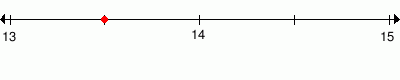131/3        131/2        133/4        13.5        14 2.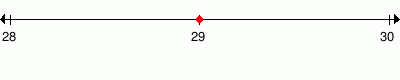291/2        29        292/3        30

### Complexity=4, Mode=1

Choose one or more answers that represent the point in the number line

 1.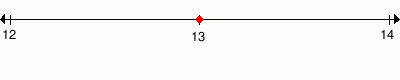131/2        14        132/3        13 2.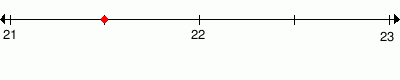211/2        212/3        211/4        21.5        22

### Complexity=6, Mode=2

Choose one or more answers that represent the point in the number line

 1.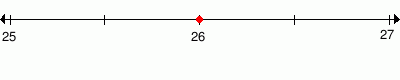261/3        26        261/2        27 2.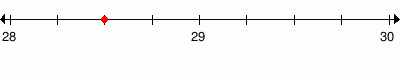281/2        29        28.5        281/4

### Complexity=8, Mode=2

Choose one or more answers that represent the point in the number line

 1.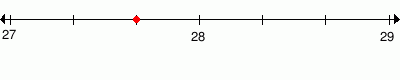271/3        271/4        272/3        27.25        28 2.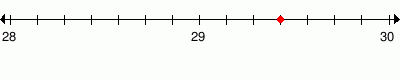293/7        296/7        292/3        297/9        297/8

### Complexity=10, Mode=3

Choose one or more answers that represent the point in the number line

 1.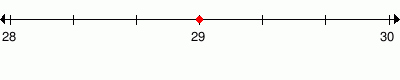2710/5        30        292/3        291/3        29 2.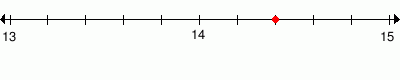142/5        143/5        14.4        143/4

### Complexity=2, Mode=1

Choose one or more answers that represent the point in the number line

1131/3        131/2        133/4        13.5        14
Solution
13 1/2
The whole number is 13 because the point is between 12 and 14
The numerator is 1 because the point is 1 notch to the right.
13.5 is decimal value of 131/2
13.5 = 13 + 5/10
= 131/2
2291/2        29        292/3        30
Solution
29
The whole number is 29 because the point is at 29.

### Complexity=4, Mode=1

Choose one or more answers that represent the point in the number line

1131/2        14        132/3        13
Solution
13
The whole number is 13 because the point is at 13.
2211/2        212/3        211/4        21.5        22
Solution
21 1/2
The whole number is 21 because the point is between 20 and 22
The numerator is 1 because the point is 1 notch to the right.
21.5 is decimal value of 211/2
21.5 = 21 + 5/10
= 211/2

### Complexity=6, Mode=2

Choose one or more answers that represent the point in the number line

1261/3        26        261/2        27
Solution
26
The whole number is 26 because the point is at 26.
2281/2        29        28.5        281/4
Solution
28 2/4
The whole number is 28 because the point is between 27 and 29
The numerator is 2 because the point is 2 notches to the right.
The denominator is 4 because there are 4 equal parts between 28 and 29

281/2
281/2 is simple fraction of 28 2/4

28.5 is decimal value of 281/2
28.5 = 28 + 5/10
= 281/2

### Complexity=8, Mode=2

Choose one or more answers that represent the point in the number line

1271/3        271/4        272/3        27.25        28
Solution
27 2/3
The whole number is 27 because the point is between 26 and 28
The numerator is 2 because the point is 2 notches to the right.
The denominator is 3 because there are 3 equal parts between 27 and 28

2293/7        296/7        292/3        297/9        297/8
Solution
29 3/7
The whole number is 29 because the point is between 28 and 30
The numerator is 3 because the point is 3 notches to the right.
The denominator is 7 because there are 7 equal parts between 29 and 30

### Complexity=10, Mode=3

Choose one or more answers that represent the point in the number line

12710/5        30        292/3        291/3        29
Solution
29
The whole number is 29 because the point is at 29.
2710/5 if simplified equal to 29.
2710/5 = 27 + 105
= 2710/5

2142/5        143/5        14.4        143/4
Solution
14 2/5
The whole number is 14 because the point is between 13 and 15
The numerator is 2 because the point is 2 notches to the right.
The denominator is 5 because there are 5 equal parts between 14 and 15

14.4 is decimal value of 142/5
14.4 = 14 + 4/10
= 142/5